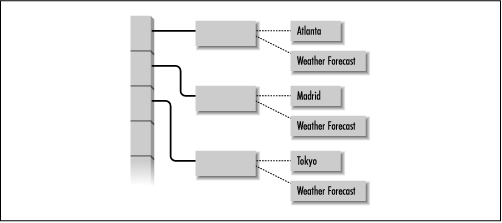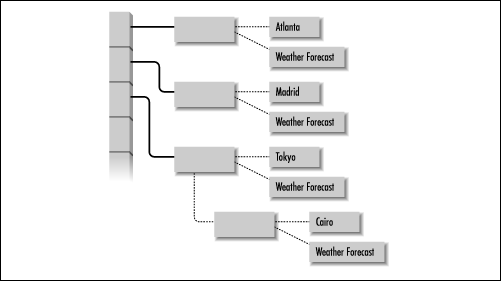start page | rating of books | rating of authors | reviews | copyrights

### 5.4 Hashtables

The Dictionary class is an abstract class that defines methods for associating key objects with value objects. Given a key, an instance of Dictionary is able to return its associated value. The Hashtable class is a concrete subclass of Dictionary that uses a data structure called a hashtable and a technique called chained hashing to allow values associated with keys to be fetched with minimal searching. You might use a Hashtable object to associate weather reports with the names of cities and towns, for example.

Before explaining hashtables or chained hashing, consider the problem of finding a key/value pair in an array that contains references to key/value pairs in no particular order. The array might look something like what is shown in Figure 5.1.

#### Figure 5.1: An array of key/value pairsSince we cannot make any assumptions about where in the array a key is to be found, the most reasonable search strategy is a linear search that starts at one end of the array and looks at each array element until it finds what it is looking for or reaches the other end of the array. For an array with just a few elements, a linear search is a reasonable strategy, but for an array with hundreds of elements it is not. If we know where in the array to look for a key, however, we can eliminate most of the searching effort. Knowing where to look for a key is the idea behind a hashtable.

With a hashtable, each key object has a relatively unique integer value that is called a hashcode. The Object class defines a hashCode() method, so every object in Java has such a method. The hashcode returned by this method computes an array index for a key object as follows:

```array.length % hashCode()
```

This array index, or hash index, stores the key/value pair in a hashtable array. If there is nothing stored at that index, the key/value pair is placed at that position in the array. However, if there is already a key/value pair at that hash index, the Hashtable stores the key/value pair in a linked list at that position in the array. This strategy for managing multiple keys with the same hash index is called chained hashing. The array for hashtable that uses this strategy might look like Figure 5.2.

#### Figure 5.2: An array of key/value pairs that uses chained hashingNow, when we want to fetch a key/value pair, all we have to do is recalculate the hash index for the key object and look at that position in the hashtable array. If the key stored at that hash index is the right key, then we have found what we are looking for by examining only one array element instead of searching. However, if the key is not the right key, all we have to do is search the items in the linked list at that position to find our key/value pair.

You can create a Hashtable object using the constructor that takes no arguments:

```Hashtable h = new Hashtable()
```

This constructor creates an empty Hashtable. There are other constructors that take parameters to allow you to tune the performance of a Hashtable object. The first parameter you can specify is the capacity of the hash table, which is the length of the array used to implement it. The longer the array, the less likely it is that multiple keys will share the same hash index. The default array length is 101. To create a Hashtable object with an array length of 1009, use the following constructor:

```Hashtable h = new Hashtable(1009);
```

The number that you choose for the array length should be a prime number. If it is not, the key/value pairs stored in the array will tend to be less evenly distributed.

The load factor of a hashtable is the ratio of the number of key/value pairs in the hashtable to the array length. A load factor of 0 means that the Hashtable is empty. As the load factor increases, so does the likelihood that multiple key/value pairs will share the same hash index. When the load factor becomes greater than 1, it means that the number of key/value pairs in a hashtable is greater than the array length, so that at least one hash index is being shared by multiple key/value pairs. Clearly, a low load factor is better than a high load factor in terms of performance. You can specify the maximum permissible load factor for a Hashtable object when you create it. For example:

```Hashtable h = new Hashtable(1009, .62);
```

If not specified, the maximum load factor for a Hashtable object is .75. When a key/value pair is added to a Hashtable that would otherwise cause the load factor to exceed the maximum value, the Hashtable performs a rehash. This means that the Hashtable creates a new array with a length one greater than double the length of the old array. It then recomputes the hash index for each key/value pair in the old array and stores each key/value pair in the new array at the new hash index. Obviously, this is an undesirable performance hit, so if you know approximately how many items you will add to a Hashtable, you should create one with an appropriate initial capacity.

After you have created a Hashtable object, you can add new key/value pairs to it, or modify the value in an existing key/value pair, by calling the put() method. The put() method takes two arguments: a reference to a key object and a reference to a value object. It first looks for a key/value pair in the hashtable with the key equal to the specified key. If there is such a key/value pair, the put() method replaces the previous value with the specified value and returns a reference to the previous value object. If, however, there is no such key/value pair, the put() method creates a new key/value pair, adds it to the hashtable and returns null. Here is a fragment of a class that uses a Hashtable to store weather forecasts.

```import java.util.Hashtable;
class WeatherForecastDictionary {
private Hashtable ht = new Hashtable(13291);
public void putForecast(String locale, WeatherForecast forecast) {
ht.put(locale, forecast);
}
...
```

The get() method returns the value associated with a given key in a Hashtable object. It takes one argument that is a reference to the key it should search for. If the get() method does not find a key/value pair with a key equal to the specified key, it returns null. Here is a method that uses the get() method to retrieve a weather forecast:

```public WeatherForecast getForecast(String locale) {
return (WeatherForecast)ht.get(locale);
}
```

The various equality tests done by a Hashtable use a given key object's equals() method. Because of the way that an object's hashCode() and equals() methods are used by the Hashtable class, it is important that if you override the definition of either of these methods, you do so in a consistent way. In other words, if two objects are considered equal by the equals() method for the class, then the hashCode() method for each object must return the same hashcode value. If that is not the case, when those objects are used as keys in a Hashtable object, the Hashtable will produce inconsistent results.

Once you have added key/value pairs to a Hashtable, you can use the keys() and elements() methods to get Enumeration objects that iterate through the key and value objects, respectively. The containsKey() method allows you to search the Hashtable for a particular key object, while contains() searches for a particular value object. The Hashtable class also defines a remove() method for removing key/value pairs from a Hashtable.